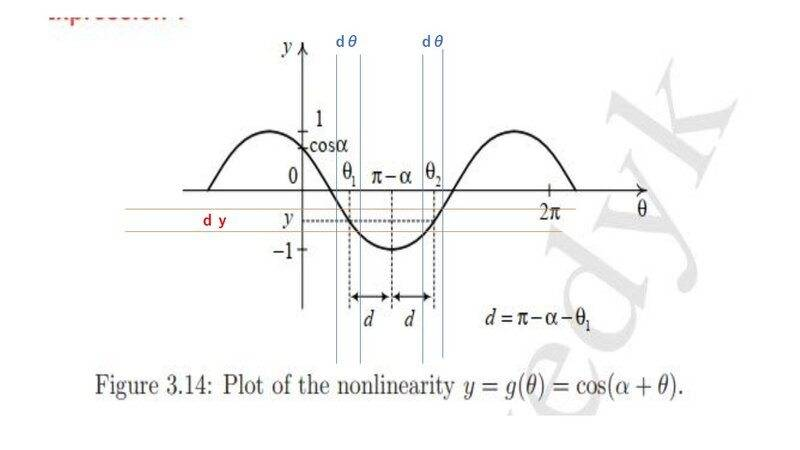# Random variable and probability density function

Homework Statement:
The problem is about continuous random variable and probability density function.
Relevant Equations:
Hi,

I was trying to solve the attached problem which shows its solution as well. I cannot understand how and where they are getting the equations 3.69 and 3.69A from.

Are they substituting the values of θ₁ and θ₂ into Expression 1 after performing the differentiation to get equations 3.70 and 3.71?

As you can see that the solution is there but I need to understand it. I'd really appreciate if you could help me with it. Thank you!

Hi-resolution image copy: https://imagizer.imageshack.com/img923/1491/lXm2aZ.jpg

#### Attachments

•prob_88.jpg
29.7 KB · Views: 23
•Delta2

anuttarasammyak
Gold Member
I am not familiar with the notation
$$f_y(y)$$
I see it is a function with variable y, but what does y in ##f_y## mean ? It seems ##f_y(y)## is defined somewhere before the problem in your text.

I am not familiar with the notation
$$f_y(y)$$
I see it is a function with variable y, but what does y in ##f_y## mean ? It seems ##f_y(y)## is defined somewhere before the problem in your text.
It means probability density function of 'f()' where "Y" is a random variable and "y" is a dummy variable, i.e. f_Y(y).

anuttarasammyak
Gold Member
Thanks. So I observe correspondence of areas for distribution of y and ##\theta## as attached.Last edited:
•PainterGuy
My apologies but I still don't get how and where they are getting the equations 3.69 and 3.69A from.

anuttarasammyak
Gold Member
$$f_y(y)dy = f_\theta(\theta) d\theta|_{\theta_1}+f_\theta(\theta) d\theta|_{\theta_2}$$ or more strictly on sign
$$f_y(y)|dy| = f_\theta(\theta) |d\theta||_{\theta_1}+f_\theta(\theta) |d\theta||_{\theta_2}$$
Divide the both sides by |dy|.

Last edited:
•PainterGuy
What is $f_\theta(\theta) d\theta$ and where does this come from? I don't see its connection with the original function y=cos(α+θ). Could you please help?

anuttarasammyak
Gold Member
$$f_\theta(\theta)\ d\theta|_{\theta_1}$$
is probability of ##\theta## to take value ## [\ \theta_1-\frac{1}{2} d\theta,\ \theta_1+ \frac{1}{2}d\theta\ ]##
It comes from the graph of y-##\theta## correspondence as I have attached.

•PainterGuy
$$f_\theta(\theta)\ d\theta|_{\theta_1}$$
is probability of ##\theta## to take value ## [\ \theta_1-\frac{1}{2} d\theta,\ \theta_1+ \frac{1}{2}d\theta\ ]##
It comes from the graph of y-##\theta## correspondence as I have attached.
Thank you but, honestly speaking, I'm still having a hard time. I think I should give it few hours, perhaps it'd make sense.

•Delta2
vela
Staff Emeritus
Homework Helper
If you have a random variable ##X##, you can have a function of that variable ##g(X)## which defines a new random variable ##Y=g(X)##. ##X## has a pdf ##f_X(x)##; similarly, the variable ##Y## has a pdf ##f_Y(y)##.

Assume for a moment that ##g(x)## monotonically increases. The function ##g## maps the interval ##(x,x+dx)## to the interval ##(y,y+dy)##, so the probability that ##X## is between ##x## and ##x+dx## should be equal to the probability ##Y## is between ##y## and ##y+dy##. In terms of the pdfs, you have
$$f_X(x)\,dx = f_Y(y)\,dy.$$ Since ##dy = g'(x)\,dx##, you get ##f_Y(y) = f_X(x)/g'(x)##.

If ##g## were a decreasing function, however, we'd run into a problem as the pdfs need to be non-negative. In that case, we should have ##f_Y(y) = -f_X(x)/g'(x)## instead. To cover both cases, we use the absolute value of ##g'(x)##.

Finally, if we drop the requirement that ##g## is a one-to-one function, then it's possible that more than one value of ##x## maps to a particular value of ##y##. In that case, we need to sum the probabilities to get
$$f_Y(y)\,dy = \sum_{g(x_i)=y} f_X(x_i)\,dx \quad \Rightarrow \quad f_Y(y) = \sum_{g(x_i)=y} \frac{f_X(x_i)}{\lvert g'(x_i) \rvert}.$$ This formula applied to this particular problem is where equation 3.69 comes from.

•PainterGuy
Thank you very much!

Since ##dy = g'(x)\,dx##, you get ##f_Y(y) = f_X(x)/g'(x)##.

In that case, we need to sum the probabilities to get
$$f_Y(y) = \sum_{g(x_i)=y} \frac{f_X(x_i)}{\lvert g'(x_i) \rvert}.$$

Isn't the last expression more general? I mean it's applicable even if g is one-to-one function or not.

Last edited:
vela
Staff Emeritus
•